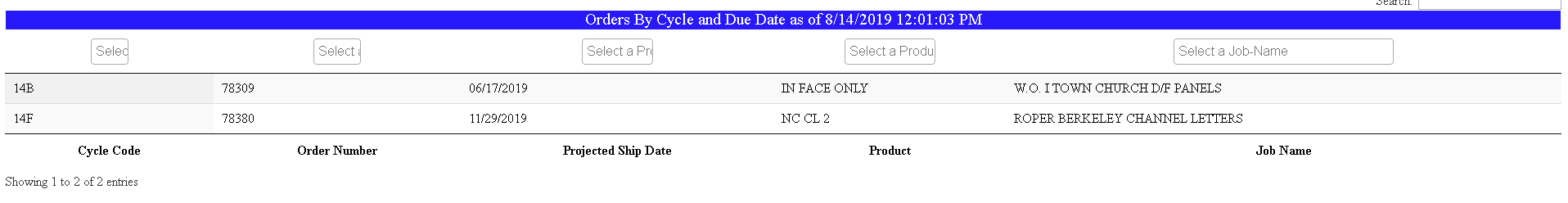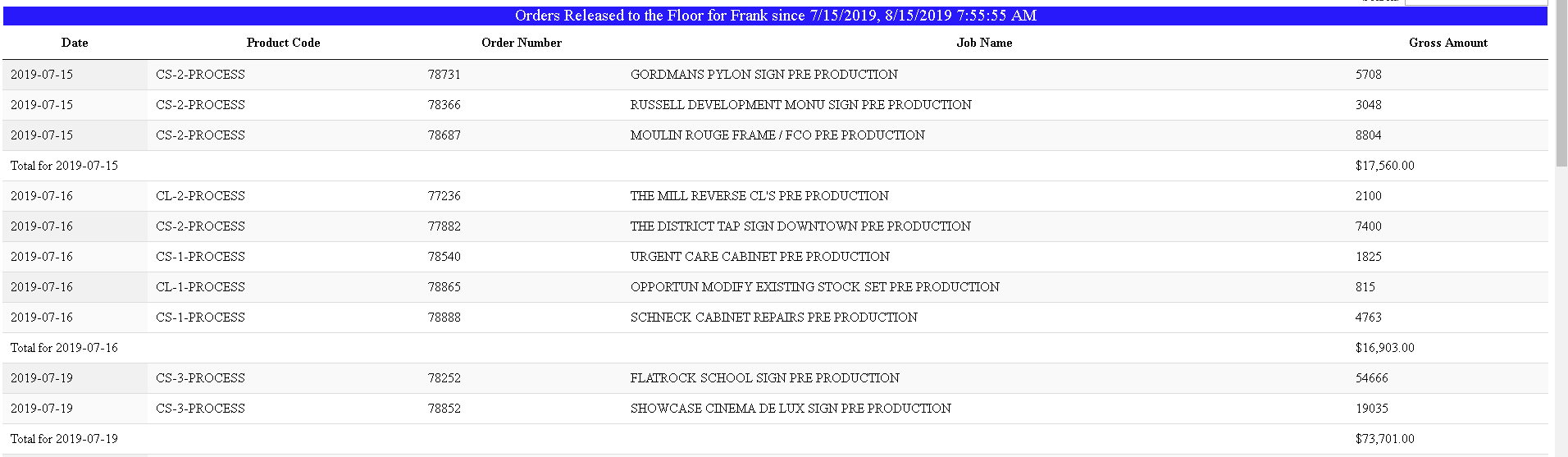# Does anyone have an example of Totals and Filtering together?

Hello,

I have two different functions that I've found here and I'd like to merge them if possible, or if someone has a working example that would be great. I'd like to be able to filter by column and then show the total on the filtered data.

Any help would be appreciated.

``````\$(document).ready(function() {
\$('#example').DataTable( {
"paging": false,
order: [[0, 'asc']],
rowGroup: {
startRender: null,
endRender: function ( rows, group ) {
var salaryAvg = rows
.data()
.pluck(4)
.reduce( function (a, b) {
return a + b.replace(/[\\$,]/g, '')*1;
}, 0);
salaryAvg = \$.fn.dataTable.render.number(',', '.', 2, '\$').display( salaryAvg );

var ageAvg = rows
.data()
.pluck(4)
.reduce( function (a, b) {
return a + b*1;
}, 0) / rows.count();

return \$('<tr/>')
.append( '<td colspan="3">Total for '+group+'</td>' )
.append( '<td/>' )
.append( '<td>'+salaryAvg+'</td>' );
},
dataSrc: 0
}
} );
} );
``````

And

``````\$(document).ready( function () {

var table = \$('#example').DataTable({
"paging":   false,
initComplete: function () {
count = 0;
this.api().columns().every( function () {
//replace spaces with dashes
title = \$(title).html().replace(/[\W]/g, '-');
var column = this;
var select = \$('<select id="' + title + '" class="select2" ></select>')
.on( 'change', function () {
//Get the "text" property from each selected data
//regex escape the value and store in array
var data = \$.map( \$(this).select2('data'), function( value, key ) {
return value.text ? '^' + \$.fn.dataTable.util.escapeRegex(value.text) + '\$' : null;
});

//if no data selected use ""
if (data.length === 0) {
data = [""];
}

//join array into string with regex or (|)
var val = data.join('|');

//search for the option(s) selected
column
.search( val ? val : '', true, false )
.draw();
} );

column.data().unique().sort().each( function ( d, j ) {
select.append( '<option value="'+d+'">'+d+'</option>' );
} );

//use column title as selector and placeholder
\$('#' + title).select2({
multiple: true,
closeOnSelect: false,
placeholder: "Select a " + title
});

//initially clear select otherwise first option is selected
\$('.select2').val(null).trigger('change');
} );
}
});
} );
``````

• Do you mean the changed total in the RowGroup'ed row? If you are able to link to the page so I can see what you are trying to do visually, that would be useful.

Thanks,
Allan

• Allan,

Thanks for responding.

Here's the two examples. The first shows how I'm filtering the data, the second how I'm totaling up dollar amounts. What I need to be able to do is on different data, is to filter the data, as there will be hundreds, if not a thousand records to start, and then show only the total for the filtered item(s).

Thanks,• So if I'm understanding correctly, you just want to move the `initComplete` function that you have for the totals into the other table with the row grouping?

Allan

• Allan,

That sounds correct.

Thanks,